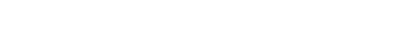# Types of Construction Project Costs – Direct and Indirect CostsReading time: 1 minute

Any construction project consists of direct and indirect costs which forms the total costs and expenses resulting from the use of principal components for implementing construction projects. Different classification of construction project costs and their features are explained below.

## Types of Construction Project Costs

Before moving into the main classification of project costs, some of the specific costs encountered in construction projects are explained below.

### 1. Fixed Cost

This is defined as the cost spent once for a particular point of time. The purchase of equipment, machinery etc comes under fixed cost assets.

### 2. Time-Related Cost

Time-related cost is the cost spend for a particular activity for a given duration. The cost spent on wages, equipment and building rents etc comes under this category.

### 3. Quantity -Proportional Cost

This type of cost will vary based on the quantities. Materials costs are examples of quantity-proportional costs. Major classification of construction projects costs are:
1. Project direct costs
2. Project Indirect Costs

Total Project Cost =  Project Direct Costs + Project Indirect Costs

## Direct Costs of Construction Project

The costs and expenses that are accountable directly on a facility, function or product are called as direct costs. In construction projects, the direct costs are the cost incurred on labor, material, equipment etc. These costs for a construction project are developed as estimates by means of detailed analysis of the contract activities, construction method, the site conditions, and resources. Different direct costs in construction projects are material costs, labor costs, subcontractor costs, and equipment costs.

## Indirect Costs of Construction Project

The costs, unlike direct costs, is not directly accountable for a particular facility, product or function. Indirect costs can be either variable or fixed. The main sections coming under indirect costs are personnel costs, security costs, and administration costs. These costs do not have a direct connection with the construction project. The indirect cost can be classified as:

The general overhead costs cannot be directly charged for a specific project. These form the costs that are used to support the overall activities of the company. The general overhead costs will include the cost of the design engineers, expenses of head-office, cost of directors and managers, schedulers etc. The general overhead expense and cost are found reasonable through continuous monitoring of the company expenses. The general overhead costs account for 2 to 5 % of the contract direct costs. The amount of the general overhead that should be allocated to a specific project equals: